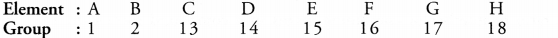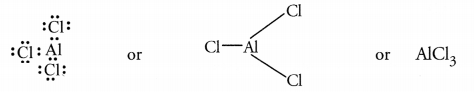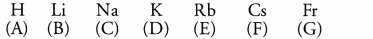# HOTS Questions for Class 10 Science Chapter 5 Periodic Classification of Elements

## HOTS Questions for Class 10 Science Chapter 5 Periodic Classification of Elements

Question 1.
Atoms of eight elements A, B, C, D, E, F, G and H have the same number of electronic shells but different number of electrons in their outermost shell. It was found that elements A and G combine to form an ionic compound. This compound is added in a small amount to almost all vegetable dishes during cooking Oxides of elements A and B are basic in nature while those of E and F are acidic. The oxide of D is almost neutral. Based on the above information answer the following questions :

1. To which group or period of the periodic table do the listed elements belong ?
2. What would be the nature of compound formed by a combination of elements B and F ?
3. Which two elements could definitely be metals ?
4. Which one of the eight elements is most likely to be found in gaseous state at room temperature ?
5. If the number of electrons in the outermost shell of elements C and G be 3 and 7 respectively, write the formula of the compound formed by the combination of C and G.

The clue for the answer is given by the compound which is added to almost all vegetables during cooking. It is sodium chloride (NaCl). Both these elements belong to third period :

1. All the elements belong to third period. They have three shells (K, L, M) and the number of electrons vary from 1 to 8. These belong to different groups which are listed.2. The element ‘B’ is Ca and ‘F’ is S. They combine to form CaS. It is an ionic compound.
3. The elements A, B are definite metals because the elements present in group 1 and 2 are all metals.
4. The element H is a noble gas element. It is most likely to be found in the gaseous state at room temperature.
5. The electronic configuration of the element C(Z = 13) is 2, 8, 3 while that of G(Z = 17) is 2, 8, 7. The formula of the compound formed by their combination is CG3. It is actually AlCl3 and is formed as a result of electron sharing.More Resources

Question 2.
The following table shows the position of six elements A, B, C, D, E and F in the period table.

 Groups Periods I 2 3 to 12 13 14 15 16 17 18 2. A B C 3. D E F

Using the above table answer the following questions :
(a) Which element will form only covalent compounds ?
(b) Which element is a metal with valency 2 ?
(c) Which element is a non-metal with valency of 3 ?
(d) Out of D and E, which one has more atomic radius and why ?
(e) Write a common name for the family of elements C and F.
(a) The element ‘E’ present in group 14 is a non-metal. Its name is silicon (Si) and the compounds of the element are only covalent.
(b) The element ‘D’ present in group 2 is a metal known as magnesium (Mg). It exhibits valency 2 in its compounds.
(c) The elements ‘B’ present in group 15 is a non-metal. It is nitrogen (N) and exhibits valency 3 in its compounds.
(d) The element ‘D’ has more atomic radius than the element ‘E’ as the atomic size decreases along a period.
(e) The elements ‘C’ and ‘F’ present in group 18 belong to a family known as noble gases.

Question 3.
Two elements X and Y belong to group 1 and 2 respectively in the same period. Compare them with respect to :
(a) the number of valence electrons
(b) valency
(c) metallic character
(d) size of the atoms
(e) formulae of their oxides and chlorides.
(a) The valence electrons present in element X (group 1) and element Y (group 2) are 1 and 2 respectively.
(b) The valency of the element X is one while that of the element Y is two.
(c) Metallic character decreases along a period. This means that the element X is more metallic as compared to element Y.
(d) Atomic size decreases along a period. As a result, the element Y has a smaller size than the element X.
(e) For element X : oxide (X2O), chloride (XCl).
For element Y : oxide (YO) and chloride (YCl2).

Question 4.
Atoms of seven elements A, B, C, D, E, F and G have a different number of electronic shells but have the same number of electrons in their outermost shells. The elements A and C combine with chlorine to form an acid and common salt respectively. The oxide of element A is liquid at room temperature and is a neutral substance while the oxides of the remaining six elements are basic in nature. Based on the above information, answer the following questions given ahead :

1. What could the element A be ?
2. Will elements A to G belong to the same period or same group of the periodic table ?
3. Write the formula of the compound formed by the reaction of the element A with oxygen.
4. Show the formation of the compound by a combination of element C with chlorine with the help of electronic structure.
5. What would be the ratio of number of combining atoms in a compound formed by the combination element A with carbon ?
6. Which one of the given elements is likely to have the smallest atomic radius ?

Since all the seven elements have same number of electrons in their outer most shells, this means that they belong to the same group. From the available information, it becomes clear that the group is of alkali metals (group 1). Hydrogen (A) is also included. The elements present are;1. The element A’ is hydrogen (H)
2. The elements A’ to ‘G’ belong to the same group and not same period
3. The formula of compound is H2O
4. Compound between ‘C’ and chlorine (Cl) is sodium chloride (NaCl) formed by the transference of one electron.5. The compound formed between A (H) and carbon is methane (CH4). In this the two elements are present in the ratio of 1 : 4
6. The element A (hydrogen) has the smallest atomic radius since it is the first element of the group.

Hope given HOTS Questions for Class 10 Science Chapter 5 Periodic Classification of Elements helpful to you.

If you have any doubts, please comment below. We try to provide online math tutoring for you.